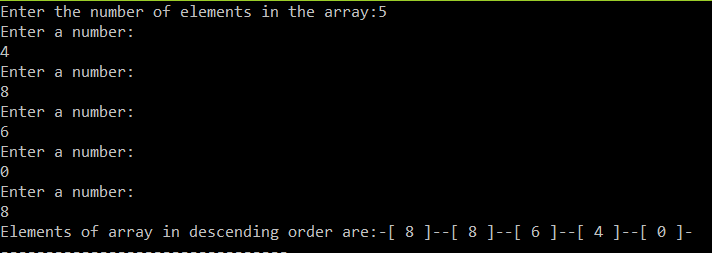# Program to sort the elements of an array in descending order in C language

The program below sort the elements of an array in descending order. It uses malloc function to allocate the space for number of elements that user enters. The code is pretty forward. With sizeof(), the program is taking the size of the n variable that’s taking the value from the user and with that value malloc is allocation that much space in the array pointer variable that is *arr. The below code has been executed in Dev c++.

#### Code:

```#include<stdio.h>
#include<stdlib.h>

void main(){
int *arr,temp,i,j,n;
printf("Enter the number of elements in the array:");

scanf("%d",&n);
arr=(int*)malloc(sizeof(int)*n);

for(i=0;i<n;i++){
printf("Enter a number:\n");
scanf("%d",&arr[i]);

}
for(i=0;i<n;i++){
for(j=i+1;j<n;j++){
if(arr[i]<arr[j]){
temp=arr[i];
arr[i]=arr[j];
arr[j]=temp;
}
}
}
printf("Elements of array in descending order are:");
for(i=0;i<n;i++){
printf("-[ %d ]-",arr[i]);
}

}
```

OUTPUT:This site uses Akismet to reduce spam. Learn how your comment data is processed.